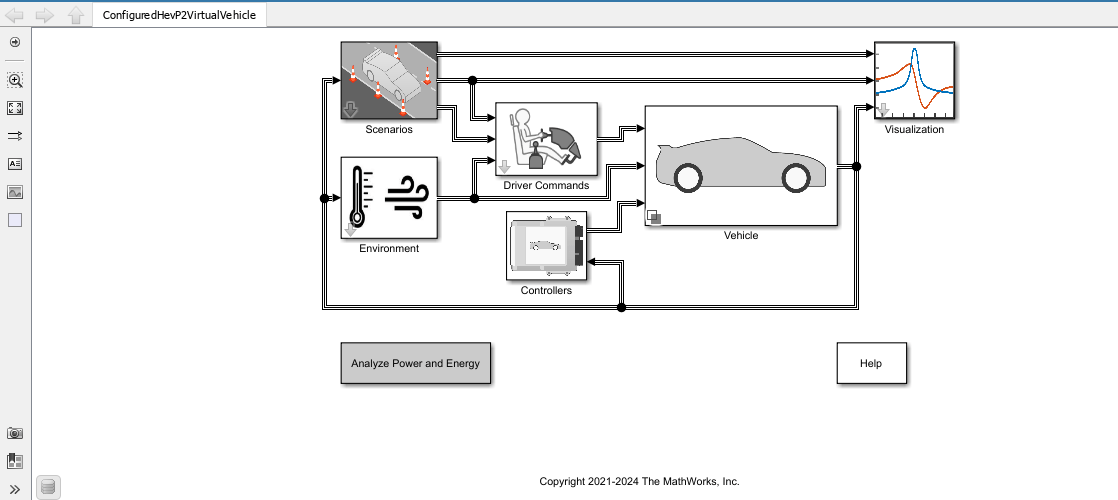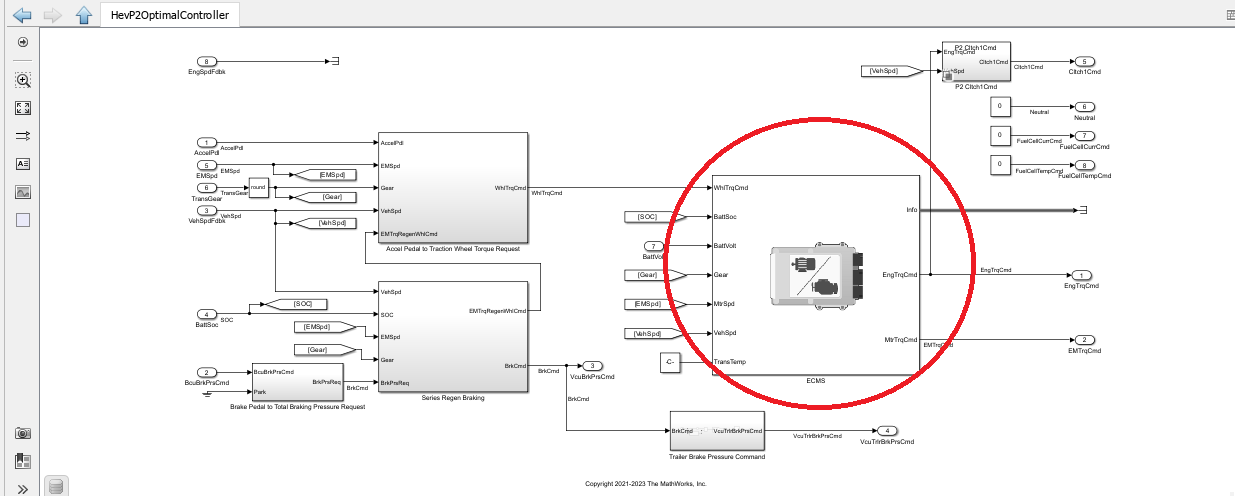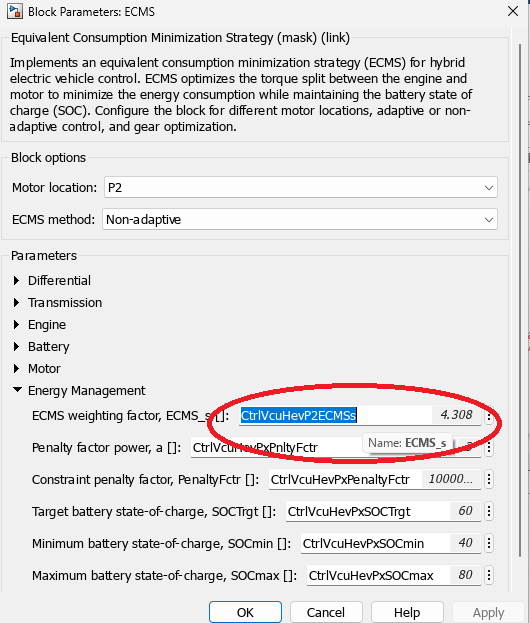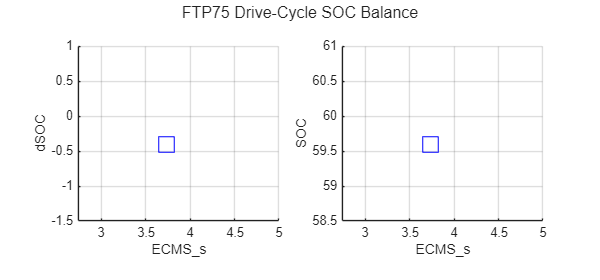# Calibrate ECMS Block for Objective Hybrid Vehicle Fuel Economy Assessment

When you compare fuel economy changes in a hybrid vehicle drive cycle, you must account for the amount of energy stored in the vehicle battery. Otherwise, net battery charging or depletion during the drive cycle can artificially inflate or deflate the fuel economy estimate for the drive cycle. Powertrain Blockset allows you to simulate the fuel economy, performance, and emissions of hybrid electric vehicles with standard hybrid powertrain architectures P0, P1, P2, P3, and P4.

Powertrain Blockset uses an industry-standard equivalent consumption minimization strategy (ECMS) to optimize the fuel economy of hybrid reference applications by optimally trading off internal combustion engine (ICE) use versus electric motor use during a given drive-cycle.

An ECMS block in the hybrid controller of each hybrid reference application optimizes trading off engine and motor use during the cycle. The ECMS block has a parameter in its block mask called ECMS_s that you can set so that the hybrid battery state of charge (SOC) at the end of a given drive cycle is the same as the SOC at the beginning of the drive cycle.

This script optimizes the ECMS_s parameter automatically. The script runs a drive-cycle simulation, measures starting and ending SOC, and repeats the process until the difference between starting and ending SOC is minimized.

During the optimization process, an optimization plot indicates the progress of the optimizer toward net zero change in SOC over the given driven cycle for the chosen vehicle configuration.

Note that the optimization process may take more than one hour to complete. After optimization completes, the ECMS_s parameter for net zero change in cycle SOC is stored in the model workspace of the Optimal Control Model Reference.#### ECMS is in HEV Reference Application Controllers#### ECMS Block Helps Achieve Optimal Fuel Economy Results### ECMS Calibration Script

Use this code to calibrate the ECMS_s block parameter for net zero change in battery SOC for any of the Powertrain Blockset Hybrid Reference Applications P0, P1, P2, P3, and P4.

### Choose HEV Reference Application and Run Optimization

```% Open a given reference application pxName="P2"; % HEV powertrain architecture configuration: P0,P1,P2,P3,P4 eval("autoblkHev"+pxName+"Start"); %Start a Px Reference Application SOCinit = 0.85; % initial SOC, unit is in [0, 1] ECMS_s=Calibrate_ECMS_s(pxName,SOCinit); %Run ECMS_s optimization and return optimal ECMS_s for net zero change in SOC over the drive-cycle``````Elapsed time is 420.625345 seconds. Search converged. ECMS_s parameter updated in model. ECMS_s = 4.760604, SOCEndTrg = 85.000000, SOC_end = 85.413778 ```

### Execute ECMS_s Calibration Function

```function ECMS_s=Calibrate_ECMS_s(pxName,SOCinit) % calibration_ecms_script battMdl = "BattHev"+pxName; % battery model - for SOC sweep mdlName="Hev"+pxName+"ReferenceApplication"; % Hev model ctrlMdl="Hev"+pxName+"OptimalController"; % Controller model SOCEndTrg = SOCinit*100; maxIter=4; % Set up optimization progress plot window size and position x0=600; y0=1040; width=600; height=280; tic load_system(mdlName); load_system(ctrlMdl); load_system(battMdl); blk = mdlName + "/" + "Drive Cycle Source"; m = get_param(blk, 'MaskObject'); ECMS_CurrentCycle = m.Parameters(1,1).Value; % Current cycle setting. mdlWks = get_param(ctrlMdl,'ModelWorkspace'); % find model workspace % get current ECMS_s value ECMS_obj = getVariable(mdlWks,'ECMS_s'); if isnumeric(ECMS_obj); ECMS_CurrentValue = ECMS_obj; end if ~isnumeric(ECMS_obj); ECMS_CurrentValue = ECMS_obj.Value; end % extract initial value/name of ECMS tuning parameter p = Simulink.Mask.get(ctrlMdl+"/ECMS"); baseParamName=p.getParameter("ECMS_s").Value; battChrgMaxValue_obj = getVariable(get_param(battMdl,'modelworkspace'),'BattChargeMax'); if isnumeric(battChrgMaxValue_obj); battChrgMaxValue = battChrgMaxValue_obj; end if ~isnumeric(battChrgMaxValue_obj); battChrgMaxValue = battChrgMaxValue_obj.Value; end % set to a temp name set_param(ctrlMdl+"/ECMS",'ECMS_s',"ECMS_s_tune") save_system(mdlName,[],'SaveDirtyReferencedModels','on'); save_system(ctrlMdl,[],'SaveDirtyReferencedModels','on'); % Enable SOC recording x1_handles = get_param(mdlName+"/Visualization/Rate Transition1",'PortHandles'); x1 = x1_handles.Outport(1); Simulink.sdi.markSignalForStreaming(x1,'on'); % arrays used to store ECMS_s and SOC_end values xc = zeros (3,1); yc = zeros (3,1); % plot showing the ECMS_s and SOC_end subplot (1,2,1); set(gcf,'position',[x0,y0,width,height]) xlabel({'ECMS\_s',' '}) ylabel('dSOC') hold on; subplot (1,2,2); xlabel('ECMS\_s') ylabel('SOC') hold on; sgtitle([ECMS_CurrentCycle ' Drive-Cycle SOC Balance']); % set model workspace variables in = ModelSetVariable (mdlName, battChrgMaxValue, SOCinit,battMdl, SOCEndTrg, ctrlMdl); % get initial 3 ECMS_s points and SOC_end values [x, y, ECMS_s, SOC_end, solution_found] = dSOC_generate_starting_points (in, ECMS_CurrentValue, SOCEndTrg); if ~solution_found for i = 1 : maxIter % solve second order equation to get z = ECMS_s corresponds to y = SOCEndTrg ```

`$\begin{array}{l}SOCEndTrg=SO{C}_{init}=a{x}^{2}+bx+c,\phantom{\rule{0.5em}{0ex}}\text{where}\phantom{\rule{0.5em}{0ex}}a,b,c\phantom{\rule{0.5em}{0ex}}\text{satisfies}\phantom{\rule{0.5em}{0ex}}{y}_{i}=a{x}_{i}^{2}+b{x}_{i}+c,\phantom{\rule{0.5em}{0ex}}1\le i\le 3.\phantom{\rule{0.5em}{0ex}}\text{with}\\ \phantom{\rule{0.5em}{0ex}}{x}_{1}={\text{ECMS}}_{s}^{\left(1\right)},{y}_{1}=SO{C}_{end}\left({\text{ECMS}}_{s}^{\left(1\right)}\right),\phantom{\rule{0.5em}{0ex}}{x}_{2}={\text{ECMS}}_{s}^{\left(2\right)},\phantom{\rule{0.5em}{0ex}}{y}_{2}=SO{C}_{end}\left({\text{ECMS}}_{s}^{\left(2\right)}\right),\phantom{\rule{0.5em}{0ex}}{x}_{3}={\text{ECMS}}_{s}^{\left(3\right)},\phantom{\rule{0.5em}{0ex}}{y}_{3}=SO{C}_{end}\left({\text{ECMS}}_{s}^{\left(3\right)}\right).\end{array}$`

``` [z] = Second_order_roots (x, y, SOCEndTrg); in = in.setVariable("ECMS_s_tune", z); [SOC_end, dSOC] =dSOCsim_v1(in); subplot (1,2,1); plot(z,dSOC,'bs','MarkerSize',15) grid on hold on subplot (1,2,2); plot(z,SOC_end,'bs','MarkerSize',15) grid on hold on if (abs(SOC_end - SOCEndTrg) <= 1) % check if a solution is found ECMS_s = z; x(1) = ECMS_s; y(1) = SOC_end; solution_found = 1; break; end xc(1:3) = x (1:3); yc(1:3) = y (1:3); x(1) = z; y(1) = SOC_end; % since z and SOC_end are new points, we use them and put into x(1), y(1) [yb, II] = sort(yc,'ascend'); % sort the array for processing xb = xc(II); % begin the process to pick other two points from the original 3 point % xb(1:3), yb(1:3) if (y(1) > SOCEndTrg) % y(1)> SOCEndTrg, we need to pick other points < SOCEndTrg if ((yb(2) < SOCEndTrg) && (SOCEndTrg < yb(3))) % yb(2) < SOCEndTrg, pick yb(2) x(2) = xb(2); y(2) = yb(2); if (abs(yb(1)-SOCEndTrg) < abs(yb(3)-SOCEndTrg)) % pick last point from xb(1), and xb(3), according to shortest distance to SOCEndTrg x(3) = xb(1); y(3) = yb(1); else x(3) = xb(3); y(3) = yb(3); end end if ((yb(1) < SOCEndTrg) && (SOCEndTrg < yb(2))) % yb(1) < SOCEndTrg, pick yb(1) x(2) = xb(1); y(2) = yb(1); if (abs(yb(2)-SOCEndTrg) < abs(yb(3)-SOCEndTrg)) % pick last point from xb(2) and xb(3), according to shortest distance to SOCEndTrg x(3) = xb(2); y(3) = yb(2); else x(3) = xb(3); y(3) = yb(3); end end end if (y(1) < SOCEndTrg) % y(1)< SOCEndTrg, we need to pick other points > SOCEndTrg if ((yb(2) < SOCEndTrg) && (SOCEndTrg < yb(3))) % yb(3) > SOCEndTrg, pick yb(3) x(2) = xb(3); y(2) = yb(3); if (abs(yb(1)-SOCEndTrg) < abs(yb(2)-SOCEndTrg)) % pick last point from xb(1) and xb(2), according to shortest distance to SOCEndTrg x(3) = xb(1); y(3) = yb(1); else x(3) = xb(2); y(3) = yb(2); end end if ((yb(1) < SOCEndTrg) && (SOCEndTrg < yb(2))) % yb(2) > SOCEndTrg, pick this point x(2) = xb(2); y(2) = yb(2); if (abs(yb(1)-SOCEndTrg) < abs(yb(3)-SOCEndTrg)) % pick last point from xb(1) and xb(3), according to shortest distance to SOCEndTrg x(3) = xb(1); y(3) = yb(1); else x(3) = xb(3); y(3) = yb(3); end end end end y_abs = abs(y-SOCEndTrg); [yb, II] = sort(y_abs,'ascend'); xb = x(II); ECMS_s = xb (1); end hold off; toc; if solution_found fprintf ('Search converged. ECMS_s parameter updated in model. \n'); fprintf ('ECMS_s = %f, SOCEndTrg = %f, SOC_end = %f \n',ECMS_s, SOCEndTrg, SOC_end); else fprintf ('Search failed to converge. An approximate ECMS_s is updated in the model. \n'); fprintf ('Refer to the Troubleshooting section of the example page for recommendations. \n'); end ECMS_s_tune = ECMS_s; % reset model Simulink.sdi.markSignalForStreaming(x1,'off'); load_system(ctrlMdl) set_param(ctrlMdl+"/ECMS",'ECMS_s',baseParamName) save_system(mdlName,[],'SaveDirtyReferencedModels','on'); % update model and sim hws = get_param(ctrlMdl, 'modelworkspace');% get the workspace hws.assignin('ECMS_s',ECMS_s); save_system(ctrlMdl,[],'SaveDirtyReferencedModels','on'); open_system(mdlName); load_system(battMdl); battChrgMaxValue_obj = getVariable(get_param(battMdl,'modelworkspace'),'BattChargeMax'); if isnumeric(battChrgMaxValue_obj); battChrgMaxValue = battChrgMaxValue_obj; end if ~isnumeric(battChrgMaxValue_obj); battChrgMaxValue = battChrgMaxValue_obj.Value; end in = in.setVariable('BattCapInit', battChrgMaxValue*SOCinit,'Workspace',battMdl); in = in.setVariable('SOCTrgt', SOCEndTrg,'Workspace',ctrlMdl); in = in.setVariable('SOCmin', max(SOCEndTrg-20,20.5),'Workspace',ctrlMdl); in = in.setVariable('SOCmax', min(SOCEndTrg+20,100),'Workspace',ctrlMdl); set_param(ctrlMdl+"/ECMS",'ECMS_s',"ECMS_s"); save_system(battMdl); save_system(ctrlMdl); end function in = ModelSetVariable (mdlName, battChrgMaxValue, SOCinit, battMdl, SOCEndTrg, ctrlMdl) in = Simulink.SimulationInput(mdlName); in = in.setVariable('BattCapInit', battChrgMaxValue*SOCinit,'Workspace',battMdl); in = in.setVariable('SOCTrgt', SOCEndTrg,'Workspace',ctrlMdl); in = in.setVariable('SOCmin', max(SOCEndTrg-20,20.5),'Workspace',ctrlMdl); in = in.setVariable('SOCmax', min(SOCEndTrg+20,100),'Workspace',ctrlMdl); end %% function [x_out, y_out, ECMS_s, SOC_end, solution_found] = dSOC_generate_starting_points (in, ECMS_CurrentValue, SOCEndTrg) x_out = zeros (3,1); y_out = zeros (3,1); x = zeros (4,1); y = zeros (4,1); solution_found = false; ECMS_s = ECMS_CurrentValue; alpha = 0.8; tol = 1; x1 = ECMS_CurrentValue; % use current ECMS_s value as the starting point in = in.setVariable("ECMS_s_tune", x1); [SOC_end, dSOC] =dSOCsim_v1(in); %evaluate x1 results y1 = SOC_end; subplot (1,2,1); plot(x1,dSOC,'bs','MarkerSize',15) grid on hold on subplot (1,2,2); plot(x1,y1,'bs','MarkerSize',15) grid on hold on if (abs(y1 - SOCEndTrg) <= tol) % check if a solution found ECMS_s = x1; SOC_end = y1; solution_found = true; end if ((y1 > SOCEndTrg) && ~solution_found) x2 = x1 - ECMS_S_dis_up (x1, y1, SOCEndTrg); % use a decreased ECMS_s value as x2 in = in.setVariable("ECMS_s_tune", x2); [SOC_end, dSOC] =dSOCsim_v1(in); y2 = SOC_end; subplot (1,2,1); plot(x2,dSOC,'bs','MarkerSize',15) grid on hold on subplot (1,2,2); plot(x2,y2,'bs','MarkerSize',15) grid on hold on if (abs(y2 - SOCEndTrg) <= tol) % check if a solution found ECMS_s = x2; SOC_end = y2; solution_found = true; end if ((y2 > SOCEndTrg) && ~solution_found) % y2 is still too high y3_try = SOCEndTrg - 2; % set a lower SOC end trial to it will be easier to get y2 < SOC_EndTrg x3 = x1 + alpha*(y3_try - y1)*(x2-x1)/(y2-y1); % use x1, y1, x2, y2 to fit a linear function```

$x={x}_{1}+\alpha \frac{y-{y}_{1}}{{y}_{2}-{y}_{1}}×\left({x}_{2}-{x}_{1}\right)$ such that $x={x}_{1}\phantom{\rule{0.5em}{0ex}}\text{at}\phantom{\rule{0.5em}{0ex}}y={y}_{1},\phantom{\rule{0.5em}{0ex}}\text{and}\phantom{\rule{0.5em}{0ex}}x=\alpha {x}_{2}+\left(1-\alpha \right){x}_{1}\phantom{\rule{0.5em}{0ex}}\text{at}\phantom{\rule{0.5em}{0ex}}y={y}_{2}$

``` dx = ECMS_S_dis_up (x2, y2, SOCEndTrg); % get a pre-defined decrease value x3 = min (x3, x2 - dx); % upper limit for x3 x3 = max (x3, x2 - 2*dx); % lower limit for x3 in = in.setVariable("ECMS_s_tune", x3); % set the x3 value as ECMS_s [SOC_end, dSOC] =dSOCsim_v1(in); % evaluate y3 = SOC_end; subplot (1,2,1); plot(x3,dSOC,'bs','MarkerSize',15) grid on hold on subplot (1,2,2); plot(x3,y3,'bs','MarkerSize',15) grid on hold on if (abs(y3 - SOCEndTrg) <= tol) % check if a solution is found ECMS_s = x3; SOC_end = y3; solution_found = true; end if ~solution_found x_out(1) = x1; x_out(2) = x2; x_out(3) = x3; y_out(1) = y1; y_out(2) = y2; y_out(3) = y3; % output 3 points end end if ~solution_found if ((y2 > SOCEndTrg) && (y3 >= SOCEndTrg)) % if y3 is still greater than SOCEndTrg, lower it again for it_again = 1 : 10 [x(4)] = Second_order_roots (x_out, y_out, SOCEndTrg - 2); % decrease again dx = ECMS_S_dis_up (x_out (3), y_out (3), SOCEndTrg); % standard decrease x(4) = max (x(4), x_out (3) - dx); % make it higher than the standard increase x(4) = min (x(4), x_out (3) - 2*dx); % limit the increase to 2 times the standard in = in.setVariable("ECMS_s_tune", x(4)); [SOC_end, ~] = dSOCsim_v1(in); ECMS_s = x(4); y(4) = SOC_end; x_out (1) = x_out (2); y_out (1) = y_out (2); x_out (2) = x_out (3); y_out (2) = y_out (3); x_out (3) = x(4); y_out (3) = SOC_end; if (abs(y(4) - SOCEndTrg) <= tol); break; end if (SOC_end <= SOCEndTrg); break; end end if (abs(y(4) - SOCEndTrg) <= tol) ECMS_s = x(4); SOC_end = y(4); solution_found = true; end end end if ((y2 < SOCEndTrg) && ~solution_found) % y2 is lower than SOCEndTrg while y1 is greater than SOCEndTrg x_min = min (x1, x2); x_max = max (x1, x2); y_min = min(y1, y2); y_max = max (y1, y2); % sort the min and max w_min = abs (y_min - SOCEndTrg) / (abs(y_min - SOCEndTrg) + abs(y_max - SOCEndTrg)); % weighting factor x3 = (1-w_min) * x_min + w_min * x_max; % x3 is a weighted interpolation between x1 and x2 in = in.setVariable("ECMS_s_tune", x3); [SOC_end, dSOC] =dSOCsim_v1(in); y3 = SOC_end; subplot (1,2,1); plot(x3,dSOC,'bs','MarkerSize',15) grid on hold on subplot (1,2,2); plot(x3,y3,'bs','MarkerSize',15) grid on hold on if (abs(y3 - SOCEndTrg) <= tol) ECMS_s = x3; SOC_end = y3; solution_found = true; end end if ~solution_found x_out(1) = x1; x_out(2) = x2; x_out(3) = x3; y_out(1) = y1; y_out(2) = y2; y_out(3) = y3; % output 3 points end end if ((y1 < SOCEndTrg) && ~solution_found) % y1 < SOCEndTrg x2 = x1 + ECMS_S_dis_up (x1, y1, SOCEndTrg); % use a higher ECMS_s value as x2 in = in.setVariable("ECMS_s_tune", x2); [SOC_end, dSOC] =dSOCsim_v1(in); y2 = SOC_end; subplot (1,2,1); plot(x2,dSOC,'bs','MarkerSize',15) grid on subplot (1,2,2); plot(x2,y2,'bs','MarkerSize',15) grid on hold on if (abs(y2 - SOCEndTrg) <= tol) ECMS_s = x2; SOC_end = y2; solution_found = true; end if ((y2 < SOCEndTrg) && ~solution_found) % x2 is not high enough y3_try = SOCEndTrg + 2; % set a higher SOC target ```

$x={x}_{1}+\alpha \frac{y-{y}_{1}}{{y}_{2}-{y}_{1}}\left({x}_{2}-{x}_{1}\right),\phantom{\rule{0.5em}{0ex}}x={x}_{1},\phantom{\rule{0.5em}{0ex}}\text{at}\phantom{\rule{0.5em}{0ex}}y={y}_{1},x=\alpha {x}_{2}+\left(1-\alpha \right){x}_{1}\phantom{\rule{0.5em}{0ex}}\text{at}\phantom{\rule{0.5em}{0ex}}y={y}_{2}$,

``` x3 = x1 + alpha*(y3_try - y1)*(x2-x1)/(y2-y1); % use x1, y1, x2, y2 as a linear prdeiction dx = ECMS_S_dis_up (x2, y2, SOCEndTrg); % standard increase x3 = max (x3, x2 + dx); % lower limit for x3 x3 = min (x3, x2 + 2*dx); % upper limit for x3 in = in.setVariable("ECMS_s_tune", x3); [SOC_end, dSOC] =dSOCsim_v1(in); y3 = SOC_end; subplot (1,2,1); plot(x3,dSOC,'bs','MarkerSize',15) grid on subplot (1,2,2); plot(x3,y3,'bs','MarkerSize',15) grid on if (abs(y3 - SOCEndTrg) <= tol) ECMS_s = x3; SOC_end = y3; solution_found = true; end if ~solution_found x_out(1) = x1; x_out(2) = x2; x_out(3) = x3; y_out(1) = y1; y_out(2) = y2; y_out(3) = y3; % output 3 points end end if ~solution_found if ((y2 < SOCEndTrg) && (y3 <= SOCEndTrg)) % y3 is still not high enough for it_again = 1 : 10 [x(4)] = Second_order_roots (x_out, y_out, SOCEndTrg + 2); % increase again dx = ECMS_S_dis_up (x_out (3), y_out (3), SOCEndTrg); % standard increase x(4) = max (x(4), x_out (3) + dx); % make it higher than the standard increase x(4) = min (x(4), x_out (3) + 2*dx); % limit the increase to 2 times the standard in = in.setVariable("ECMS_s_tune", x(4)); [SOC_end, dSOC] = dSOCsim_v1(in); ECMS_s = x(4); y(4) = SOC_end; x_out (1) = x_out (2); y_out (1) = y_out (2); x_out (2) = x_out (3); y_out (2) = y_out (3); x_out (3) = x(4); y_out (3) = SOC_end; if (SOC_end >= SOCEndTrg); break; end if (abs(y(4) - SOCEndTrg) <= tol); break; end end subplot (1,2,1); plot(x(4),dSOC,'bs','MarkerSize',15) grid on subplot (1,2,2); plot(x(4),y(4),'bs','MarkerSize',15) grid on if (abs(y(4) - SOCEndTrg) <= tol) ECMS_s = x(4); SOC_end = y(4); solution_found = true; end end end if ((y2 > SOCEndTrg) && ~solution_found) % y2 is good x_min = min (x1, x2); x_max = max (x1, x2); y_min = min(y1, y2); y_max = max (y1, y2); % get min and max for x1, x2, y1, y2 w_min = abs (y_min - SOCEndTrg) / (abs(y_min - SOCEndTrg) + abs(y_max - SOCEndTrg)); % weighted factor x3= (1-w_min) * x_min + w_min * x_max; % get weighted interpolation between x1, and x2 in = in.setVariable("ECMS_s_tune", x3); [SOC_end, dSOC] =dSOCsim_v1(in); y3 = SOC_end; subplot (1,2,1); plot(x3,dSOC,'bs','MarkerSize',15) grid on subplot (1,2,2); plot(x3,y3,'bs','MarkerSize',15) grid on if (abs(y3 - SOCEndTrg) <= tol) ECMS_s = x3; SOC_end = y3; solution_found = true; end end if ~solution_found x_out(1) = x1; x_out(2) = x2; x_out(3) = x3; y_out(1) = y1; y_out(2) = y2; y_out(3) = y3; % output three points end end end function [SOC_end, dSOC]=dSOCsim_v1(in) evalc('out=sim(in)'); %Simulate suppressing build info if isempty(out.ErrorMessage) SOC=out.logsout.getElement('Battery SOC').Values.Data; dSOC=SOC(end)-SOC(1); SOC_end = SOC(end); else dSOC=NaN; end end function dx = ECMS_S_dis_up (ECMS_s, y, SOC_target) % function computes standard dx = increase/decrease in ECMS_s % the dx is proportional to the distance between SOC_end (y) and SOC_Target % also, dx is proportional to ECMS_s, a, y, ff can be adjusted ff = 0.05; a = 0.015; c = 3.5; b = ECMS_s / c; % ECMS_s effect if (ECMS_s < c-0.1); b = 1; end dx = a + b * abs(y-SOC_target) * ff; end function [z] = Second_order_roots (x, y, y_tar)```

```d1 = y(1) / (x(1) - x(2)) / (x(1) - x(3)); d2 = y(2) / (x(2) - x(1)) / (x(2) - x(3)); d3 = y(3) / (x(3) - x(1)) / (x(3) - x(2)); AA = (d1 + d2 + d3); BB = - (d1 * (x(2) + x(3)) + d2 * (x(1) + x(3)) + d3 * (x(1) + x(2))); CC = d1 * x(2) * x(3) + d2 * x(1) * x(3) + d3 * x(1) * x(2) - y_tar; z1 = (-BB + sqrt (BB*BB - 4 * AA * CC)) / 2 / AA; z = real(z1); end```